# Difference Between Isotonic Hypotonic and Hypertonic

## Main Difference – Isotonic vs Hypotonic vs Hypertonic

A solution is a homogeneous liquid mixture of two or more components. A solution is made by dissolving a solute in a solvent. There are three types of solutions grouped based on their concentrations. The concentration of a solution is the amount of solute present in a unit volume of the solution. The concentration of a solution determines its osmotic pressure; the minimum pressure required to avoid a solution flowing through a semipermeable membrane. The main difference between isotonic hypotonic and hypertonic solutions is that isotonic solutions are solutions having equal osmotic pressures and hypotonic solutions are solutions having a lower osmotic pressure whereas hypertonic solutions are solutions with a high osmotic pressure.

### Key Areas Covered

1. What is Isotonic
– Definition, Effect on Cells
2. What is Hypotonic
– Definition, Effect on Cells
3. What is Hypertonic
– Definition, Effect on Cells, Uses
4. What is the Difference Between Isotonic Hypotonic and Hypertonic
– Comparison of Key Differences

Key Terms: Concentration, Hypertonic, Hypotonic, Isotonic, Osmotic Pressure, Solutions, Turgidity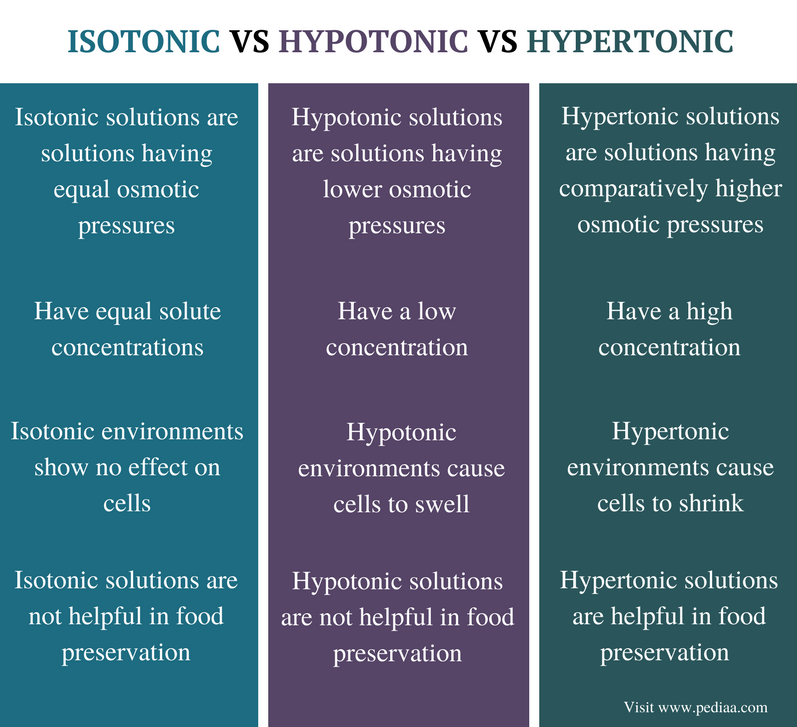## What is Isotonic

Isotonic solutions are solutions having equal osmotic pressures. This is due to the equal concentrations of solutes they have. Isotonic solutions have the same amount of solutes per unit volume of solution and the same amount of water.

When two isotonic solutions are separated from a semipermeable membrane, there is no net movement of solutes across the membrane since there is no concentration gradient between the two solutions. The rates of the movement of water from one solution to the other are equal. Therefore, the cells remain in their normal state. The shape of the cell is not changed; no swelling or shrinking occurs.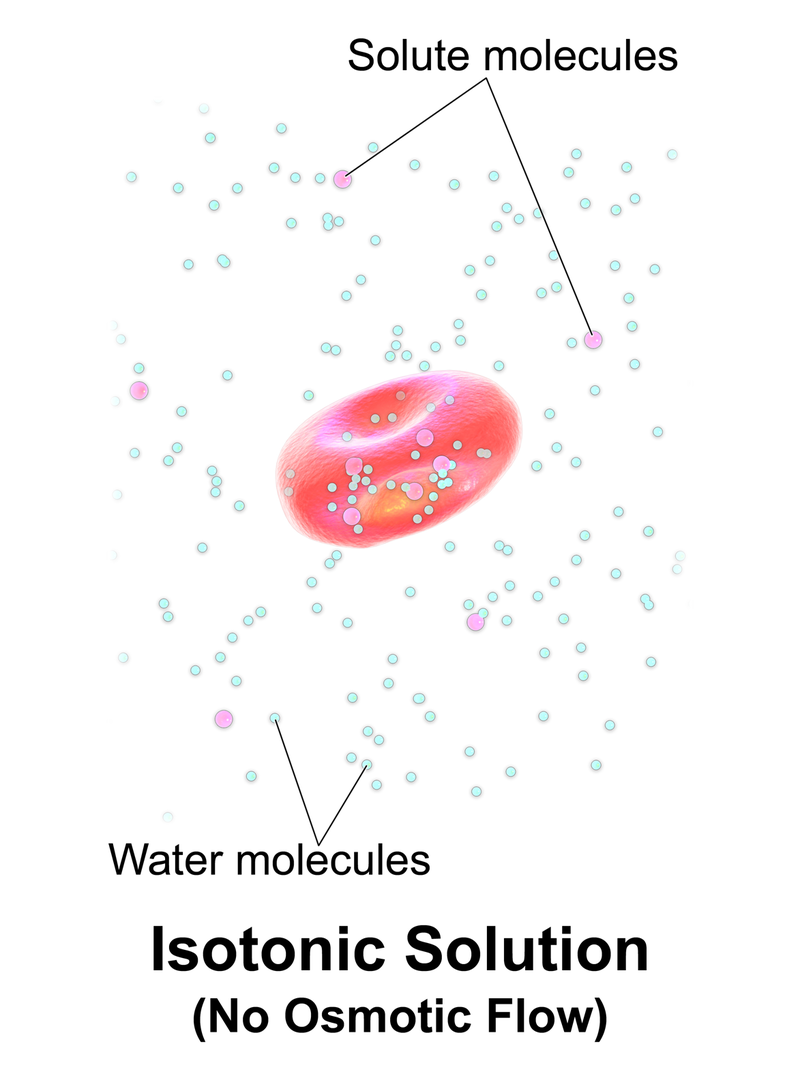Figure 1: Isotonic

Osmotic pressure is the pressure required to be applied in order to avoid this solute movement through the semipermeable membrane. Isotonic solutions have equal osmotic pressures since the rates of movement of molecules through the semipermeable membrane are equal.

Some examples for solutions that are isotonic with animal cells are given below.

## What is Hypotonic

A hypotonic solution is a solution having a lower osmotic pressure. The low osmotic pressure is a result of low solute concentration. Osmotic pressure is the pressure required to be applied in order to avoid this solute movement through the semipermeable membrane. When a hypotonic solution is separated from another solution via a semipermeable membrane, the solute movement through the membrane is less. Therefore the pressure that needs to be applied in order to stop this movement is also less.

When a cell is exposed to a hypotonic environment, the amount of water inside the cell is less than that of the hypotonic solution. This is because, in hypotonic solutions, a less amount of solutes are dissolved in a high amount of water. Then the cell swells. The internal pressure of the cell is increased and the cells may even burst.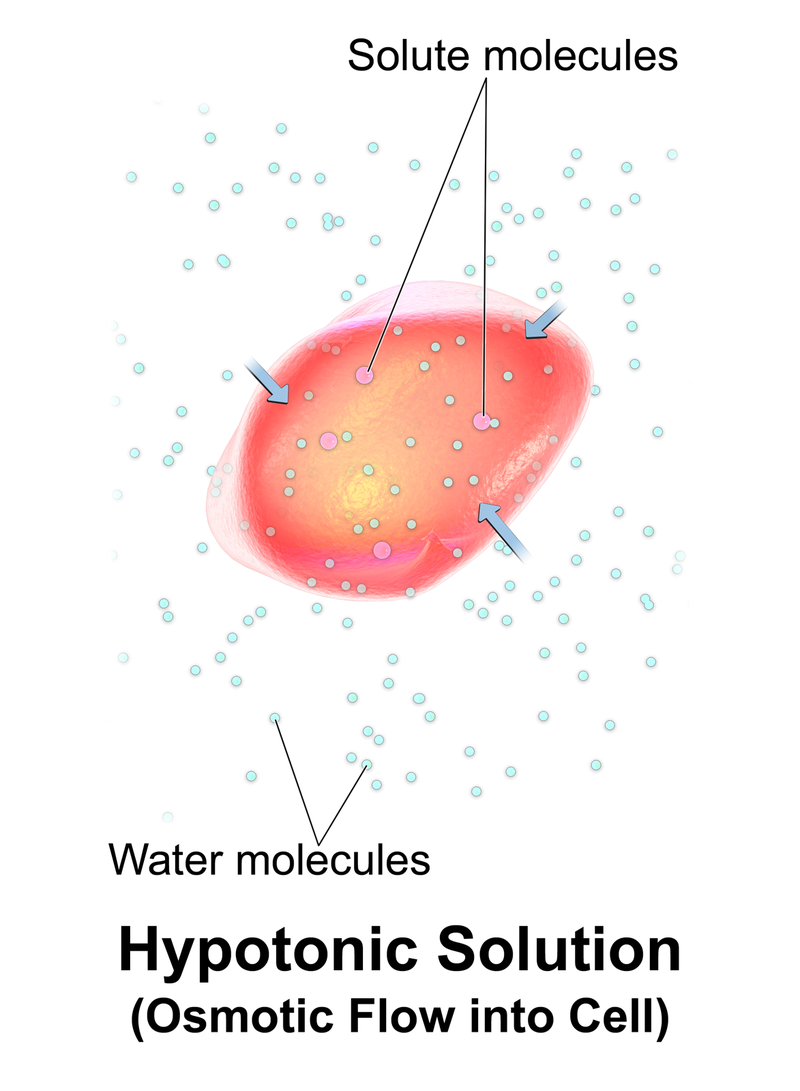Figure 2: Hypotonic

Hypotonic solutions can cause turgidity in plant cells. When water enters the plant cell, the cell swells up. As a result, the cell membrane is pushed towards the plant cell wall. The cell wall can avoid the cell bursting. This process is turgidity, or we call this swelled cell a “turgid cell”.

## What is Hypertonic

A hypertonic solution is a solution having a higher osmotic pressure when compared to other solutions. Since hypertonic solutions have higher solute concentrations, a very high pressure has to be applied in order to avoid this solution from flowing through a semipermeable membrane.

When a hypertonic solution and another solution (that is not hypertonic) are separated from a semipermeable membrane, the solutes of the hypertonic solution tends to move across the semipermeable membrane. This is because the hypertonic solution has a higher solute concentration and the solutes can move along a concentration gradient (from a high concentration to a low concentration). A semipermeable membrane is a biological or synthetic membrane that allows some molecules and ions to pass through it.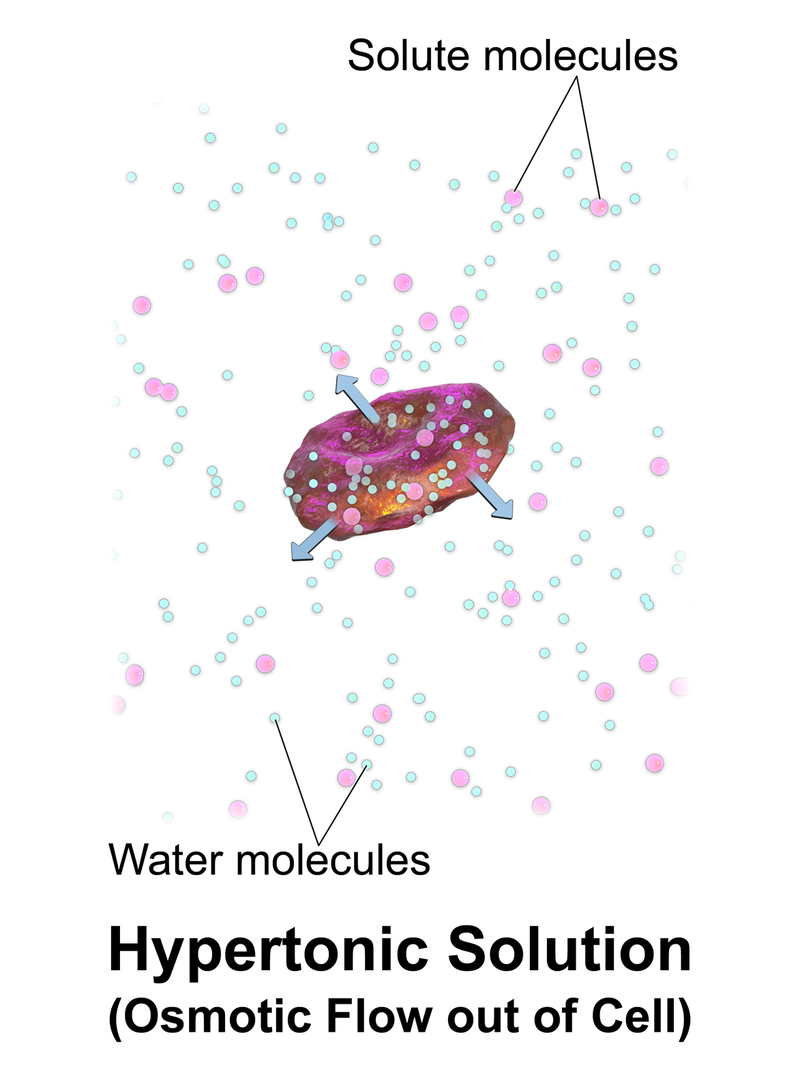Figure 3: Hypertonic

Osmotic pressure is the pressure required to be applied in order to avoid this solute movement through the semipermeable membrane. Since the concentration of a hypertonic solution is very high, the pressure required to avoid the solute movement is also high. Hence the osmotic pressure is high.

Hypertonic solutions are used to preserve food. For example, when some fruits or fish is dipped in a hypertonic salt or packaged with a hypertonic solution, it can kill microbes in the environment inside the package. This is because microbial cells have a high amount of water than solutes and the amount of water in a hypertonic solution is very low. Therefore water flows out of the cells according to the concentration gradient. The lack of water causes the shrinking of the cell and eventually kills microbes.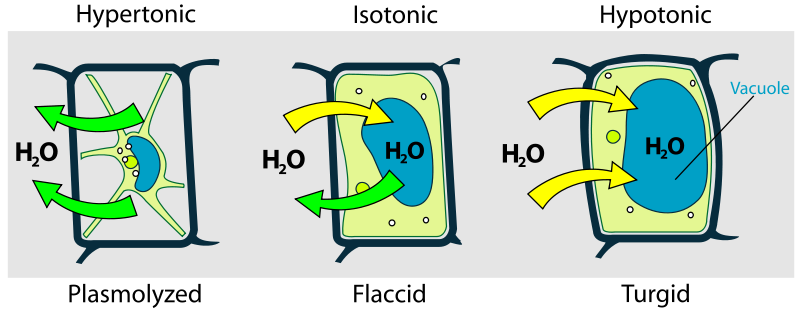Figure 1: Turgidity in Plant Cells

## Difference Between Isotonic Hypotonic and Hypertonic

### Definition

Isotonic: Isotonic solutions are solutions having equal osmotic pressures.

Hypotonic: Hypotonic solutions are solutions having lower osmotic pressures.

Hypertonic: Hypertonic solutions are solutions having comparatively higher osmotic pressures.

### Solute Concentration

Isotonic: Isotonic solutions have equal solute concentrations.

Hypotonic: Hypotonic solutions have a low concentration.

Hypertonic: Hypertonic solutions have a high concentration.

### Effect on Cells

Isotonic: Isotonic environments show no effect on cells.

Hypotonic: Hypotonic environments cause cells to swell.

Hypertonic: Hypertonic environments cause cells to shrink.

### Food Preservation

Isotonic: Isotonic solutions are not helpful in food preservation.

Hypotonic: Hypotonic solutions are not helpful in food preservation.

Hypertonic: Hypertonic solutions are helpful in food preservation since they kill microbes in the food package.

### Conclusion

Tonicity is the relative concentration of solutes dissolved in a solution which determines the direction and extent of the movement of molecules across a semipermeable membrane. There are three types of solutions based on the tonicity; isotonic solutions, hypertonic solutions and hypotonic solutions. The main difference between isotonic hypotonic and hypertonic solutions is that isotonic solutions are solutions having equal osmotic pressures while hypotonic solutions are solutions having a lower osmotic pressure and hypertonic solutions are solutions with a high osmotic pressure.

##### References:

1. Helmenstine, Ph.D. Anne Marie. “What Is Does Hypertonic Mean?” ThoughtCo, Available here.
2. Dewi Sivasamy Follow. “The effects of hypotonic, hypertonic and isotonic.” LinkedIn SlideShare, 26 Feb. 2013, Available here.
3. “Cells in Hypotonic Solutions.” Pearson – The Biology Place, Available here.

##### Image Courtesy:

1. “Blausen 0685 OsmoticFlow Isotonic” By Blausen.com staff (2014). “Medical gallery of Blausen Medical 2014”. WikiJournal of Medicine 1 (2). DOI:10.15347/wjm/2014.010. ISSN 2002-4436. – Own work (CC BY 3.0) via Commons Wikimedia
2. “Blausen 0684 OsmoticFlow Hypotonic” By Blausen.com staff (2014). “Medical gallery of Blausen Medical 2014”. WikiJournal of Medicine 1 (2). DOI:10.15347/wjm/2014.010. ISSN 2002-4436. – Own work (CC BY 3.0) via Commons Wikimedia
3. “Blausen 0683 OsmoticFlow Hypertonic” By Blausen.com staff (2014). “Medical gallery of Blausen Medical 2014”. WikiJournal of Medicine 1 (2). DOI:10.15347/wjm/2014.010. ISSN 2002-4436. – Own work (CC BY 3.0) via Commons Wikimedia
4. “Turgor pressure on plant cells diagram” By LadyofHats (Public Domain) via Commons Wikimedia